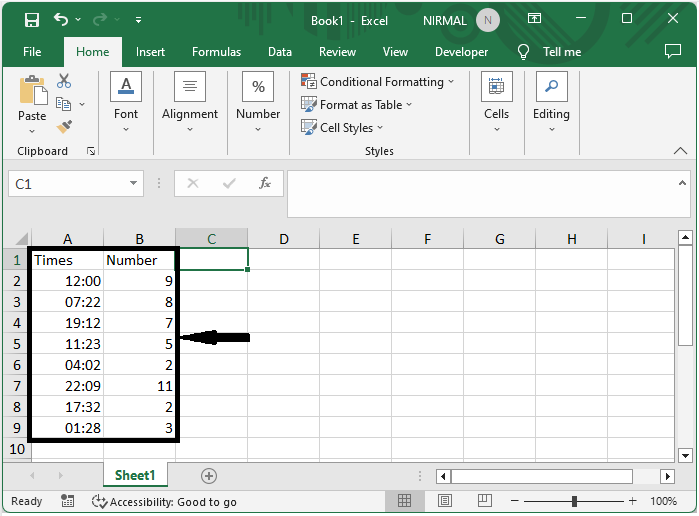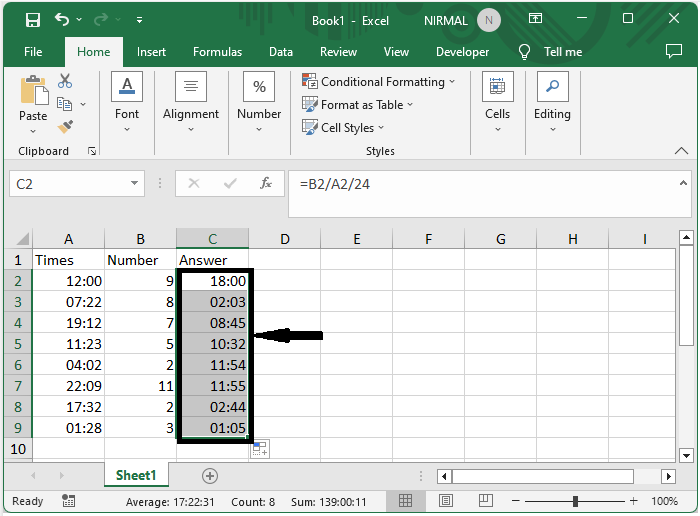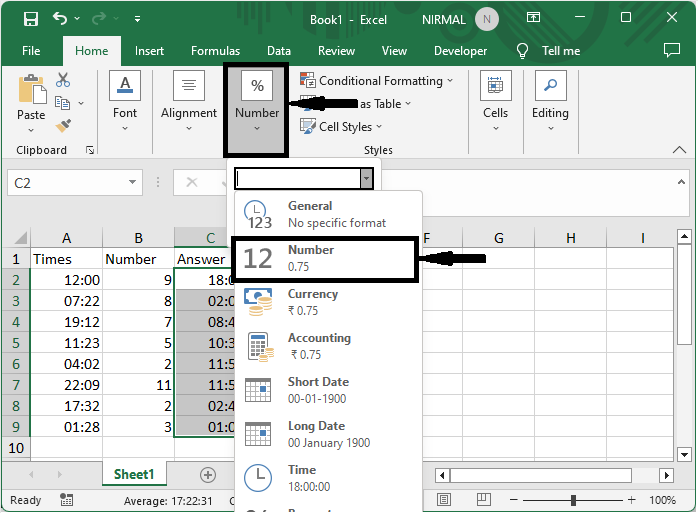# How to Divide Time by a Number (Distance) in Excel?

Microsoft Excel is a robust spreadsheet programme that lets you do a variety of computations and operations on your data. Divide time by a number is a typical operation that can be beneficial in a variety of contexts, such as calculating average time per distance or finding the time required to accomplish a unit of distance. In this article, we will look at various methods for dividing time by a number in Excel. We will cover both fundamental and advanced approaches, allowing you to select the approach that best meets your needs. Whether you are a novice or a seasoned Excel user, this video will provide you with the information and abilities you need to properly divide time by a number in your spreadsheets.

## Divide Time by a Number (Distance)

Here we will first divide the columns, then convert the result into a number. So let us see a simple process to learn how you can divide time by a number (distance) in Excel.

### Step 1

Consider an Excel sheet where you have two lists, one containing number and the other with times, similar to the below image.First, click on an empty cell, in our case, cell C2, and enter the formula as =B2/A2/24. Click enter, then drag using the autofill handle. In the formula, B2 is a cell containing numbers, and A2 is a list of times.

Empty cell > Formula > Enter.### Step 2

Then select the range of cells, click on the custom under number group, and select a number to complete the task.

Select cells > Home > Custom > Number.## Conclusion

In this tutorial, we have used a simple example to demonstrate how you can divide time by a number (distance) in Excel to highlight a particular set of data.

Updated on: 12-Jul-2023

1K+ Views